# Python | Pandas Index.union()

Python is a great language for doing data analysis, primarily because of the fantastic ecosystem of data-centric python packages. Pandas is one of those packages and makes importing and analyzing data much easier.

Pandas` Index.union()` function form the union of two Index objects and sorts if possible. The function follows behaves like a standard set union operation. The function can also find the union of categorical data.

Syntax: Index.union(other)

Parameters :
other : Index or array-like

Returns : union : Index

Example #1: Use `Index.union()` function to find the union of two indexes.

 `# importing pandas as pd ` `import` `pandas as pd ` ` `  `# Creating the first index ` `idx1 ``=` `pd.Index([``10``, ``20``, ``18``, ``32``]) ` ` `  `# Creating the second index ` `idx2 ``=` `pd.Index([``21``, ``10``, ``30``, ``40``, ``50``]) ` ` `  `# Print the first Index ` `print``(idx1) ` ` `  `# Print the second Index ` `print``(``"\n"``, idx2) `

Output :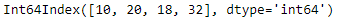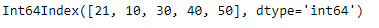Let’s find the union of these two indexes

 `# perform set union of the two indexes ` `idx1.union(idx2) `

Output :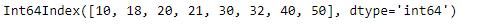The function has found the union of these two indexes.

Example #2: Use `Index.union()` function to perform set union operation on the given two indexes. Index lables are of string type.

 `# importing pandas as pd ` `import` `pandas as pd ` ` `  `# Creating the first index ` `idx1 ``=` `pd.Index([``'Harry'``, ``'Mike'``, ``'Arther'``, ``'Nick'``], ` `                                    ``name ``=``'Student'``) ` ` `  `# Creating the second index ` `idx2 ``=` `pd.Index([``'Alice'``, ``'Bob'``, ``'Rachel'``, ``'Tyler'``, ``'Louis'``], ` `                                            ``name ``=``'Winners'``) ` ` `  `# Print the first Index ` `print``(idx1) ` ` `  `# Print the second Index ` `print``(``"\n"``, idx2) `

Output :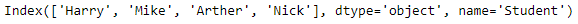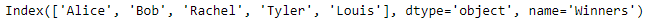Let’s find the union of these two indexes.

 `# find union of two indexes ` `idx1.union(idx2) `

Output :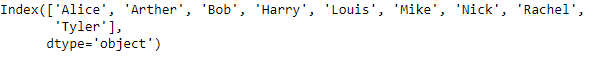The function has returned a new index that contains the result of the set union of the idx1 and idx2.

My Personal Notes arrow_drop_upCheck out this Author's contributed articles.

If you like GeeksforGeeks and would like to contribute, you can also write an article using contribute.geeksforgeeks.org or mail your article to contribute@geeksforgeeks.org. See your article appearing on the GeeksforGeeks main page and help other Geeks.

Please Improve this article if you find anything incorrect by clicking on the "Improve Article" button below.

Article Tags :

Be the First to upvote.

Please write to us at contribute@geeksforgeeks.org to report any issue with the above content.